Open in App
Not now

# Types of Forces

• Last Updated : 21 Feb, 2021

Force can be defined as a push or a pull action on an object. For any force applied there is a change in motion, state, shape, size, etc. of an object. The force has both magnitude and direction, as it is a vector quantity. It can be measured using a spring balance by placing the object at its hook end.

Forces are either long-range or short-range order. Electrostatic forces are long-range forces as well as the gravitational force is also long-range force.

The ‘rest’ state of an object is considered to be the zero speed, as-

1. An object cannot move by itself.
2. An object cannot change its speed by itself.
3. An object cannot change its direction by itself.
4. An object cannot change by itself.
5. A force may make an object move from rest.
6. A force may change the speed of a moving object.
7. A force may change the direction of a moving object.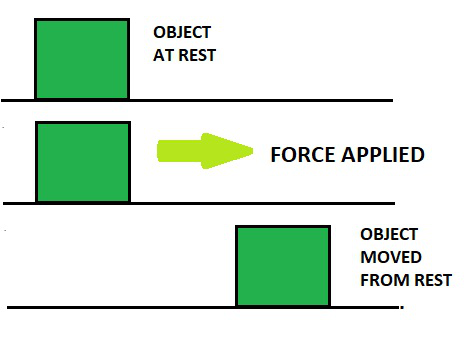Two forces applied one the same direction add up to give the resultant force and those which are applied in opposite directions the resultant force is in the direction of greater force applied.

The SI unit of force is Newton (N).

The formula of force is given by

F = ma

Where

F = Force acting on body

M = Mass of body

a = Acceleration of the body

From Newton’s second law of motion 1N force is a force that generates an acceleration of 1ms-2 in a body of mass 1kg.

1N = 1kg × 1ms-2

1N = 1 kgms-2

Some other systems of units in common use are the cgs(centimetre-gram-second) and the British systems. In the cgs system, the force has a unit as dyne, and it is equivalent to the g cms-2. As 1kg=1000 g and 1ms-2=100 cms-2, it follows that 1N =105 dyne. A dyne is a small unit, which is roughly equal to the weight of a cubic millimetre of water. (A newton as we know, on the other hand, is about the weight of a half cup of water taken).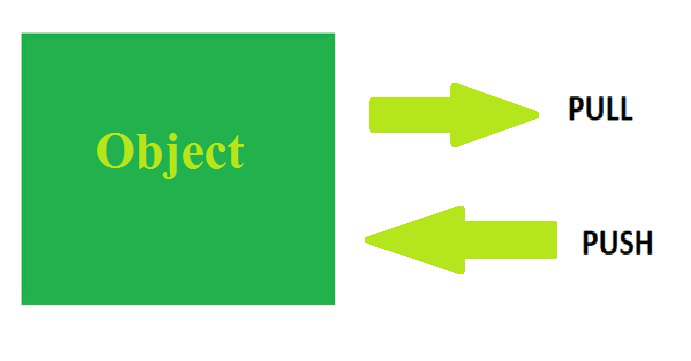## Types of Forces

There are two types of forces based on the surface of contact between the object and the surface:

### Contact force

It consists of two types of forces:

• Muscular force
• Frictional force

### Non-contact force

It consists of three types of forces:

• Electrostatic force
• Gravitational force
• Magnetic force

## Contact force

A contact force is any force that requires a contact to occur or in simple words, we can say that it arises during the motion of one object over the surface of another. Contact forces are generated only when in contact and are responsible for the most visible actions between macroscopic collections of matter. Pushing a car up an inclined surface or kicking a football across a room or in a playground or pushing any block from one place to another place or shifting large blocks from one place to another are some everyday examples where contact forces are at work.

There are two types of contact forces:

1. Frictional force
2. Muscular force

### Frictional force

Friction is a force between two surfaces that are sliding, rolling or dragging across each other. For example, If we try to push an object like book along the floor, friction makes this difficult. Frictional force or opposing force always works in the direction opposite to the direction in which the object is moving, or trying to move. Friction always slows the speed of a moving object down. The amount of frictional force exerted depends on the materials of which the two surfaces are made up of. As we increase the extent of roughness in the surface, more friction is produced. Friction also produces heat. When you try to rub your hands together quickly, you will feel them getting warmer.

For example:

• Frictional force is a useful force because it prevents our shoes from slipping on the floor when we walk and stops car tyres from skidding on the road.
• When you walk, friction is caused between the thread of shoes and the surface of the ground. This friction acts to grip the ground and prevent sliding on the road.
• Players use spikes in their shoes to enhance the friction between the ground and the shoes so that they don’t slip.
• Players use gloves during batting to increase the friction and decrease the chances of slipping the bat from their hand.

Sometimes we want to reduce friction.

For example:

• We use oil to reduce the friction between the moving parts inside a car engine. The oil tends to keep the surfaces apart and so that objects can flow easily. The reduction in frictional force or opposing force means there is less wear and tear on the car’s moving parts and less heat produced.
• When we want to have a smoother surface for moving a block from one room to another we use lubricants.
• When we ball during a cricket match we use some powdery substance in our hands to reduce the amount of friction.
• When we play carom we usually put some amount of powder on its surface to reduce the friction while playing.

The force of friction has the following formula:Where F= force of frictioncoefficient of friction

N= normal reaction force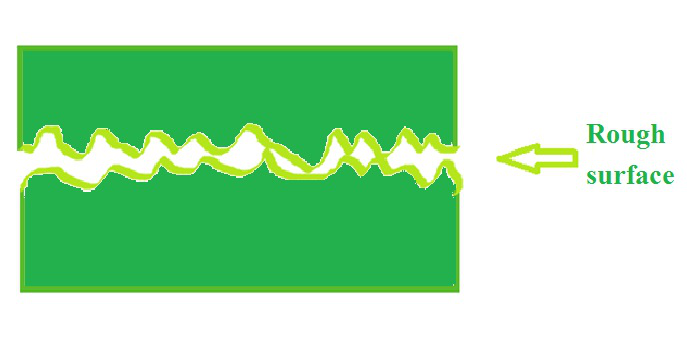### Muscular force

Our muscles exert a force on the other body when we try to lift it, bend it or in any way try to disturb its state of rest. This force exerted is called Muscular force. Many activities like lifting an object, walking on the ground, running, bending, boxing requires muscular force.

It is a contact force because muscular force can only be exerted on physical contact like walking, running, jumping, and so on or a Muscular force is called contact force because the muscles of our body are in direct contact with the object while applying force. In simple words, it is a force that results because of the action of muscles and is a contact force since there is contact between the surfaces. Muscular force is required whenever the movement of the body occurs.

For example:

• Muscular forces can also be required in Strolling, lifting, getting up from a seat, crossing a leg.
• In a game of tug of war, the team which pulls the rope with a greater force wins as the rope goes in the direction of greater force.
• Whenever we are to lift a book or any object we need to apply a force that is greater than the reaction force exerted by the object.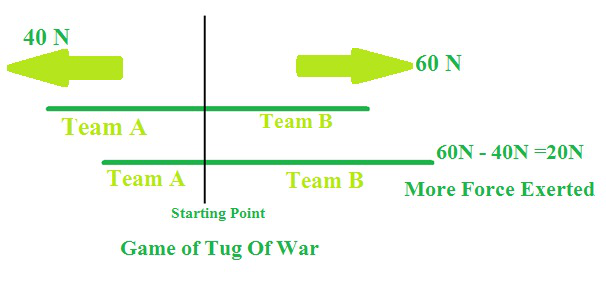## Non-contact force

A non-contact force is a force that acts on an object without coming physically in contact with it. Gravity is the most familiar non-contact force, which confers weight. In contrast, a contact force is a force applied to a body by another body that is in contact with it or in other words, Forces that arise without the contact of 2 or more objects involved. The types of non-contact forces are:

1. Gravitational force.
2. Magnetic force.
3. Electrostatic force.

### Gravitational force:

Gravity, also called gravitation, in mechanics, the universal force of attraction acting between all matters. All bodies have a weight on Earth, or downward force of gravity, proportional to their masses. Gravity is measured by the acceleration that it gives to freely falling objects. In other words, Gravitational force is a force exerted by the earth on all objects on it. Gravitational Force surrounds us everywhere we are. It is present when we are sitting, playing, walking, throwing, and everywhere. Not only Earth but any other massive celestial body exerts a gravitational pull. It can be greater or equal to or lesser than Earth’s gravitational force, but it is present to some extent. Since the bodies with a greater mass attract everything towards us hence there is a gravitational pull on anywhere we are on if it has a mass much greater than our weight.

For example:

• When a ball is thrown up, it falls to the ground because of gravitational force.
• Water always flows downwards because of gravitational force.
• When we throw an apple up it comes down because of gravitational force.
• Any fruit falls in a downward direction always it is also due to gravitational force.

It is denoted by the equation,Where, F= gravitational force

G = universal gravitational constant= product of masses of two bodiessquare of the distance between the two masses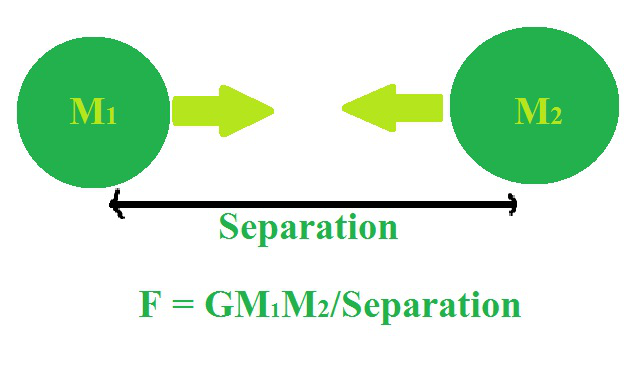### Magnetic force

Attraction or repulsion that arises between electrically charged particles because of their motion is called magnetic force. The magnetic force is a consequence of electromagnetism, one of the four fundamental forces of nature, and is caused by the motion of charges.  In simple words, two objects containing charge with the same direction of motion have a magnetic attraction force between them. If the two objects have opposite charges then they repel each other.

It is calculated by the given formula:Where, F= magnetic force

q= charge of moving particle

B= magnetic field= angle between velocity and magnetic field vectors.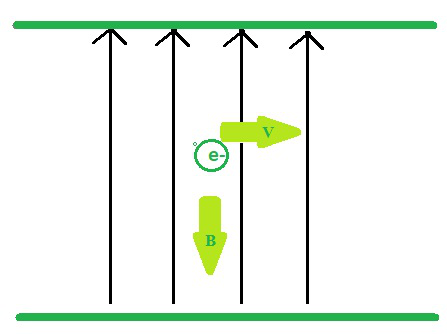### Electrostatic force

The force caused due to electric charges in an attractive and repulsive manner between the particles is called electrostatic force. The force between stationary charged bodies is conventionally known as the electrostatic force. It is also referred to as Columba’s force. In simple words, Electrostatic force is the force that exists between electrically charged particles or objects at rest.

Examples of electrostatic forces:

1. When we rub a small paper piece with the oil in our head with the help of a comb produces electrostatic force.
2. Balloons always get attracted towards another balloon when one of them is rubbed with hair.
3. When we iron silk clothes they often cling together after some time due to electrostatic charges.
4. Lightning is also considered an example of electrostatic force.

It is given by the equation,Where, F= electrostatic force of attraction

k= constant of proportionalityproduct of charges of two bodiessquare of the distance between the two bodies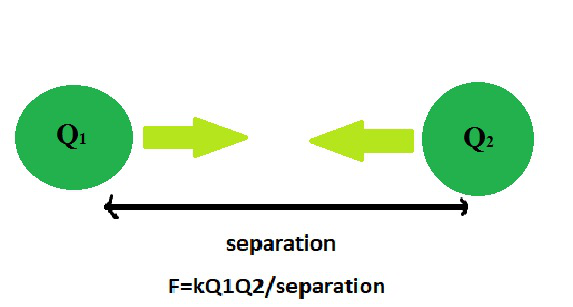### Sample Problems

Question 1. What is the frictional force on an object if the coefficient of friction is 0.50 and the normal reaction offered is 60N?

Solution:

As we know,Given, N= 60N,0.50Hence the frictional force is 30N.

Question 2. What is the coefficient of friction if the frictional force exerted is 60N and the normal reaction offered is 80N?

Solution:

As we know,Given, N= 80N

F=60NHence, the coefficient of friction is 0.75

Question 3. What is the normal reaction if the frictional force exerted is 50N and the coefficient of friction is 0.25?

Solution:

As we know,Given, F=50NHence, the normal reaction offered is 200N.

Question 4. What is the gravitational force on two bodies of masses 2kg and 4kg respectively and the universal gravitational constant (G) being with the separation between them being 4m?

Solution:

As we know,Putting the values from the question we get,On solving we getQuestion 5. What is the gravitational force on two bodies of masses 4kg and 8kg respectively and the universal gravitational constant (G) being with the separation between them being 4m?

Solution:

As we know,Putting the values from the question we get,On solving we getQuestion 6. What is the gravitational force on two bodies of masses 4kg and 6kg respectively and the universal gravitational constant (G) being with the separation between the two bodies being 2m?

Solution:

As we know,Putting the values from the question we get,On solving we get.

Question 7. What is the electrostatic force between two charges of charge 4C and 5C which are at a distance of 4m with constant of proportionality 1?

Solution:

As we know,Putting the given values from the question in the equation we getOn solving we get F=1.25N.

Question 8. What is the electrostatic force between two charges which have the product of their charges as 16 C and are at a distance of 6m with a constant of proportionality 1?

Solution:

As we know,Putting the given values from the question in the equation we getOn solving we get F=0.45N.

Question 9. What is the electrostatic force between two charges of charge (-3C) and (-6C) which are at a distance of 4m with constant of proportionality 1?

Solution:

As we know,Putting the given values from the question in the equation we getOn solving we get F=1.125N.

Question 10. What is the expression to calculate magnetic force?

Solution:

Magnetic forces can be calculated by the following equationWhere, F= magnetic force

q= charge of moving particle

B= magnetic fieldangle between velocity and magnetic field vectors.

Question 11. What is the magnetic force exerted by a charge of 3 C moving with a velocity of 6m/s in a magnetic field of 6 T and the value of sin

*** QuickLaTeX cannot compile formula:

*** Error message:
Error: Nothing to show, formula is empty


?

Solution:

Magnetic forces can be calculated by the following equationWhere we have,

q= 3C

B= 6 Tv= 6m/s

putting the values we have,Hence the magnetic force is 54N.

Question 12. What is the magnetic force exerted by a charge of 4C moving with a velocity of 8m/s in a magnetic field of 8T and the value of?

Solution:

As we know,Where we have,

q= 4C

B= 8 Tv= 8m/s

Putting the values we have,Hence, the magnetic force is 256N.

Question 13. In a game of tug of war the force applied by one team was 60N and the opponent team applied a force of 80N. Predict which team will win the game?

Solution:

The team which applied a force of 80 N will win the game because since they applied a force greater the 60 N which the other team applied.

Hence the resultant force=80-60 N =20N which is in the direction of the other team.

Question 14. If Ramesh wants to lift a dumble of weight 60 N how much force does he needs to apply to lift it?

Solution:

As we know that to lift the dumble, he needs to apply a force which is greater than 60N which can be any force greater than that. For we know that to lift an object we have to overcome the reaction force exerted by it.

Question 15. Two girls are playing the game of pushing a ball of weight 50N. One of them applied a force of 70N and the other girl applied a force of 40N. Which of them will be able to move the ball and win the game?

Solution:

As we know to push an object we need to apply a greater amount of force than its weight hence the girl who applied 70N force will win the game as she applied a greater force than the weight of object. The second girl who applied a force of 40N will lose as the force applied by her was not sufficient to overcome the force of reaction provided by the ball.

My Personal Notes arrow_drop_up